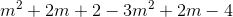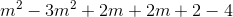## Example Questions

### Example Question #66 : Polynomials

A hockey team has 12 forwards, 6 defensemen, and 2 goalies.  When they place 3 forwards, 2 defensemen, and 1 goalie on the ice, how many of each type are on the bench, (not on the ice)?  F=Forward, D = Defensemen, G= Goalie

9 F, 4 D, 1 G

15 F, 8 D, 3 G

6 F, 2 D, 1 G

36 F, 12 D, 2 G

4 F, 3 D, 2 G

9 F, 4 D, 1 G

Explanation:

We can represent this as a subtraction of trinomials.

(12F + 6D + 2G) – (3F + 2D + 1G) = 9F + 4D + 1G.

### Example Question #67 : Polynomials

You go to the grocery store and pick upapples,peaches, andoranges. Today you had some friends over who ateapples,peaches, andoranges. Which of the following represents how many of each you now have left if= apples,= peaches, and= oranges?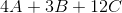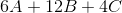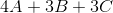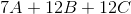Explanation:

1. Represent the situation with two sets of trinomials:

Before your friends ate the fruit: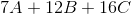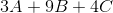2. Subtract the first trinomial from the second trinomial: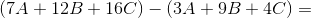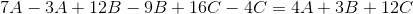### Example Question #68 : Polynomials

Choose the answer which best simplifies the following expression: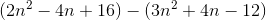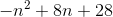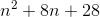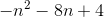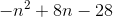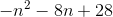Explanation:

To solve this expression, merely remove the parentheses (bearing in mind that because the second trinomial is being subtracted, it will be negative) and combine like terms: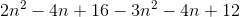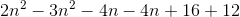### Example Question #69 : Polynomials

Choose the answer which best simplifies the following expression: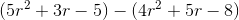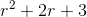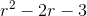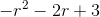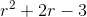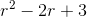Explanation:

To simplify, remove parentheses and combine like terms: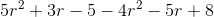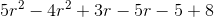### Example Question #70 : Polynomials

Choose the answer which best simplifies the following expression: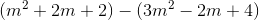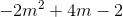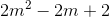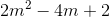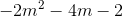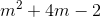Explanation:

To simplify, remove parentheses and combine like terms: